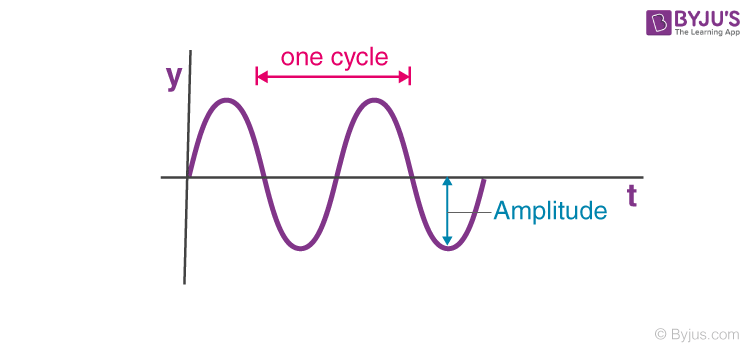# Frequency, Time Period And Angular Frequency

As we know, many forms of energy like light and sound travel in waves. A wave is defined through various characteristics like frequency, amplitude and speed. In wave mechanics, any given wave enfolds parameters like – frequency, time period, wavelength, amplitude etc. This article lets us understand and learn in detail about frequency, time period, and angular frequency.

Frequency definition states that it is the number of complete cycles of waves passing a point in unit time. The time period is the time taken by a complete cycle of the wave to pass a point. Angular frequency is angular displacement of any element of the wave per unit time.

Consider the graph shown below. It represents the displacement y of any element for a harmonic wave along a string moving in the positive x-direction with respect to time. Here, the element of the string moves up and down in simple harmonic motion.The relation describing the displacement of the element with respect to time is given as:

y (0,t) = a sin (–ωt), here we have considered the inception of from x=0

y (0,t) = -a

As we know, sinusoidal or harmonic motion is periodic in nature, i.e. the nature of the graph of an element of the wave repeats itself at a fixed duration. To mark the duration of periodicity following terms are introduced for sinusoidal waves.

Following is the table explaining other related concepts of waves:

## What is Time Period?

As shown above, in sinusoidal wave motion, the particles move about the mean equilibrium or mean position with time. The particles rise till they reach the highest point, that is, the crest, and then continue to fall till they reach the lowest point that is the trough. The cycle repeats itself in a uniform pattern. The time period of oscillation of a wave is defined as the time taken by any string element to complete one such oscillation. For a sine wave represented by the equation:
y (0, t) = -a sin(ωt)

The time period formula is given as:

 $$T=\frac{2\pi }{\omega }$$

## What is Frequency?

We define the frequency of a sinusoidal wave as the number of complete oscillations made by any element of the wave per unit of time. By the definition of frequency, we can understand that if a body is in periodic motion, it has undergone one cycle after passing through a series of events or positions and returning to its original state. Thus, frequency is a parameter that describes the rate of oscillation and vibration.

The equation gives the relation between the frequency and the period:

The relation between the frequency and the period is given by the equation:

f=1/T

For a sinusoidal wave represented by the equation:

y (0,t) = -a

The formula of the frequency with the SI unit is given as:

 Formula $$f=\frac{1}{T}=\frac{\omega }{2\pi }$$ SI unit Hertz

One Hertz is equal to one complete oscillation taking place per second.

## What is Angular Frequency?

For a sinusoidal wave, the angular frequency refers to the angular displacement of any element of the wave per unit time or the rate of change of the phase of the waveform. It is represented by ω. Angular frequency formula and SI unit are given as:

 Formula $$\omega=\frac{2\pi }{T}=2\pi f$$ SI unit rads-1

Where,

• ω = angular frequency of the wave.
• T = time period of the wave.
• f = ordinary frequency of the wave.

Here is a video demonstrating the time period, frequency and wavelength of a sound wave.

## Frequently Asked Questions – FAQs

### What is a wave?

A wave is a disturbance that travels through a medium from one location to another location.

f = 1/T

### Define Frequency.

We define the frequency of a sinusoidal wave as the number of complete oscillations made by any element of the wave per unit of time.

### Define Time Period.

The time period of oscillation of a wave is defined as the time taken by any string element to complete an oscillation.

### Define Angular Frequency.

The angular frequency refers to the angular displacement of any element of the wave per unit time or the rate of change of the waveform phase.

Test your knowledge on Period Angular Frequency

#### 1 Comment

1. thanks for the simple answer